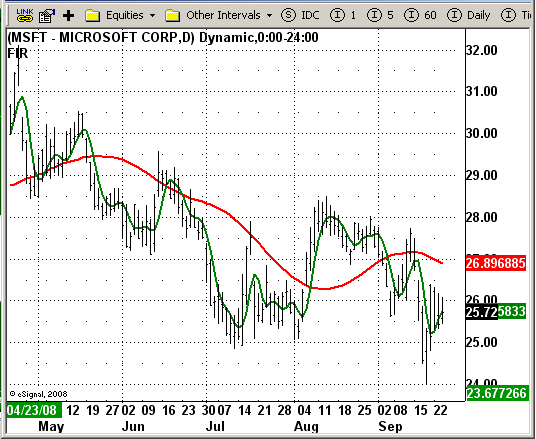# Four-Element Laguerre Filter by John Ehlers

ICE Data Services -

LaguerreFilter.efs                                                                                                           EFSLibrary - Discussion Board

File Name: LaguerreFilter.efs

Description:
Four-Element Laguerre Filter by John Ehlers

Formula Parameters:
Gamma - damping factor: 0.8

Notes:
This moving average indicator allows to avoid whipsaw trades and strike a balance
between the amount of smoothing and the amount of lag by using some modern math tools
like Laguerre transform filter. This filter is the next generation tool comparing to
either the usual MovAvg or FIR (finite impulse response) filters.

LaguerreFilter.efsEFS Code:

```/*********************************Provided By: eSignal (Copyright c eSignal),
a division of Interactive Data Corporation. 2008.
purposes only and may be modified and saved under a new file name.
eSignal is not responsible for the functionality once modified.
eSignal reserves the right to modify and overwrite this EFS file with each new release.Description:
Four-Element Laguerre Filter by John Ehlers Version: 1.0 09/24/2008Notes:
This moving average indicator allows to avoid whipsaw trades and strike a balance between
the amount of smoothing and the amount of lag by using some modern math tools like Laguerre
transform filter. This filter is the next generation tool comparing to either the usual MovAvg or
FIR (finite impulse response) filters.
Formula Parameters: Default: Gamma - damping factor 0.8**********************************/

var fpArray = new Array();
var bInit = false;

function preMain() {
setPriceStudy(true);
setStudyTitle("FIR");
setCursorLabelName("Filt", 0);
setDefaultBarFgColor(Color.red, 0);
setDefaultBarThickness(2, 0);
setCursorLabelName("FIR", 1);
setDefaultBarFgColor(Color.green, 1);
setDefaultBarThickness(2, 1);
var x = 0;
fpArray[x] = new FunctionParameter("gamma", FunctionParameter.NUMBER);
with (fpArray[x++]) {
setName("Gamma");
setLowerLimit(0.01);
setDefault(0.8);
}
}
var L0_1 = 0;
var L1_1 = 0;
var L2_1 = 0;
var L3_1 = 0;
var L0 = 0;
var L1 = 0;
var L2 = 0;
var L3 = 0;
var xhl2 = null;

function main(gamma) {
if (gamma == null) gamma = 0.8;
if (bInit == false) {
xhl2 = hl2();
bInit = true;
}
if (getBarState() == BARSTATE_NEWBAR) {
L0_1 = L0;
L1_1 = L1;
L2_1 = L2;
L3_1 = L3;
}
var Filt = 0;
var FIR = 0;
var sum = 0;
var i = 0;
L0 = (1 - gamma) * xhl2.getValue(0) + gamma * L0_1;
L1 = -gamma * L0 + L0_1 + gamma * L1_1;
L2 = -gamma * L1 + L1_1 + gamma * L2_1;
L3 = -gamma * L2 + L2_1 + gamma * L3_1;
Filt = (L0 + 2 * L1 + 2 * L2 + L3) / 6;
for (i = -3; i <= 0; i++) {
if (i == 0 || i == -3) k = 1;
else k = 2;
sum += k * xhl2.getValue(i);
}
FIR = sum / 6;
if (getCurrentBarCount() < 50) return;
return new Array(Filt, FIR);
}
```